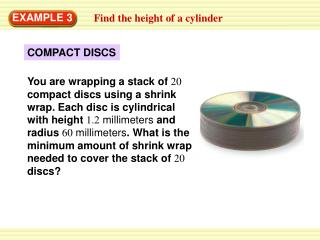DownloadDownload PresentationEXAMPLE 3

# EXAMPLE 3

Télécharger la présentation## EXAMPLE 3

- - - - - - - - - - - - - - - - - - - - - - - - - - - E N D - - - - - - - - - - - - - - - - - - - - - - - - - - -
##### Presentation Transcript

1. You are wrapping a stack of 20 compact discs using a shrink wrap. Each disc is cylindrical with height 1.2millimeters and radius 60millimeters. What is the minimum amount of shrink wrap needed to cover the stack of 20 discs? EXAMPLE 3 Find the height of a cylinder COMPACT DISCS

2. ANSWER You will need at least 31,667square millimeters, or about 317square centimeters of shrink wrap. EXAMPLE 3 Find the height of a cylinder SOLUTION The 20 discs are stacked, so the height of the stack will be 20(1.2) = 24 mm.The radius is 60millimeters. The minimum amount of shrink wrap needed will be equal to the surface area of the stack of discs. S = 2πr2 + 2πrh Surface area of a cylinder. = 2π(60)2 + 2π(60)(24) Substitute known values. ≈ 31,667 Use a calculator.

3. Find the height of the right cylinder shown, which has a surface area of 157.08square meters. EXAMPLE 4 Find the height of a cylinder SOLUTION Substitute known values in the formula for the surface area of a right cylinder and solve for the height h. S = 2πr2 + 2πrh Surface area of a cylinder.

4. ANSWER The height of the cylinder is about 7.5meters. EXAMPLE 4 Find the height of a cylinder Substitute known values. 157.08 = 2π(2.5)2 + 2π(2.5)h 157.08 = 12.5π + 5πh Simplify. Subtract 12.5π from each side. 157.08 – 12.5π = 5πh 117.81 ≈ 5πh Simplify. Use a calculator. 7.5 ≈ h Divide each side by 5π.

5. GUIDED PRACTICE for Examples 3 and 4 3. Find the surface area of a right cylinder with height 18centimeters and radius 10centimeters. Round your answer to two decimal places. SOLUTION S = 2πr2 + 2πrh Surface area of a cylinder. = 2π(60)2 + 2π(10)18 Substitute known values. = 1759.29 cm2 Use a calculator.

6. GUIDED PRACTICE for Examples 3 and 4 4. Find the radius of a right cylinder with height5 feet and surface area 208πsquare feet. SOLUTION Surface area of a cylinder. S = 2πr2 + 2πrh 208π =2π(r)2+ 2πr(5) Substitute known value. 208π = 2πr2 + 10πr Simplify. Divide 2π from each side. 104 = r2 +5r

7. ANSWER The radius of cylinder is 8feet. GUIDED PRACTICE for Examples 3 and 4 r = 8 Simplify. Use a calculator.